# An illustrated glossary of AI terminologies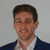##### Mickaël Wajnberg, Ph.D.
1 year ago
Share:

Artificial intelligence, machine learning, deep learning, data mining... These terms are more and more omnipresent in our daily life but often used in a confused and imprecise manner. This blog aims to help non-specialists to navigate with more confidence in the terminology used in the field of Artificial Intelligence.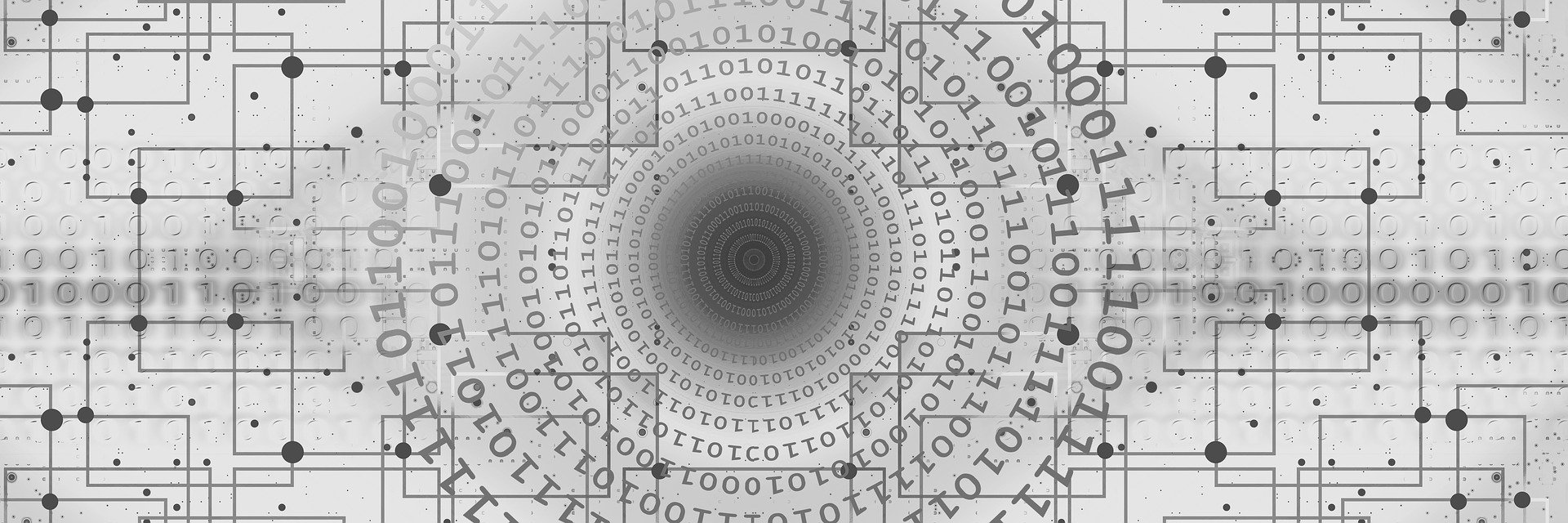## Artificial Intelligence

Artificial intelligence (AI) is defined by the Larousse online encyclopedia as the "set of theories and techniques used to create machines capable of simulating human intelligence". However, this definition only partially reflects the reality of scientists in the field. AI is a complex domain that focus its interest mainly in two main dimensions.

The first question to be asked; *is intelligence purely defined by the capacities of Homo Sapiens or in a more abstract way by rationality and logic. Then follows a question relating to the support used for this intelligence; can the result, i.e. the behavior seen from the outside, be a proof of intelligence or should we also examine the internal process of reflection?.

By crossing the two axis: (1) human vs. rational (2) behavior vs. internal process we obtain four possible definitions for the field of AI, each of which has given rise to a complete field of research.
1. Human behavior According to Alan Turing, and his test proposed in 1950, we can define an entity as artificial intelligence from the moment when a human is not able to distinguish between a communication with another human or the machine being tested. The test is as follows: we place a candidate in communication with a human entity and a machine, if the candidate cannot say with certainty which of these of these entities is the machine, the stage of artificial intelligence is reached.

2. Human thinking Here, artificial intelligence is at the crossroads of the worlds of computer science and cognitive science. The idea is not simply to observe the result given by the machine but to study from a psychological point of view the intermediate decisions taken to evaluate the general reasoning and to create a machine whose internal functioning would be similar to the human.

3. Rational thinking The goal of rational thinking AIs is to follow as closely as possible the rules of deduction that have been emerging since ancient Greece. Indeed, the most famous example is surely the syllogism "Socrates is a man, all men are mortal, therefore Socrates is mortal". However, these rules are not limited to syllogisms because real facts are rarely black and white. The most modern approaches combine formal rules (syllogisms) with probability management.

4. Rational behavior At the complete opposite of the Larousse definition, this sub-field of AI is nevertheless the most important (in terms of productivity, size of the community, number of articles published etc.). It consists in creating agents, i.e. entities that act in a rational way and this, without restriction on the way internal processes. These agents are defined to meet a specific need and, taking into account its environment and the constraints imposed, aims to maximize the result with respect to this need.

### The rational agent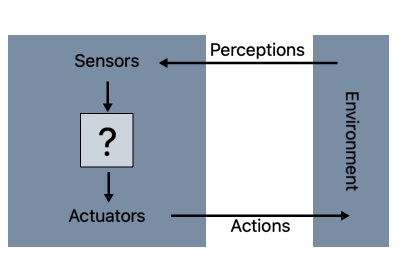Figure 1

An agent is a system that perceives its environment (virtual or not) with the help of sensors, calculates the best performance according to its constraints and objectives, and then acts.

For example, an automatic chess opponent perceives the board, makes the decision that will most likely lead to victory, then moves a piece.

### The reflex agent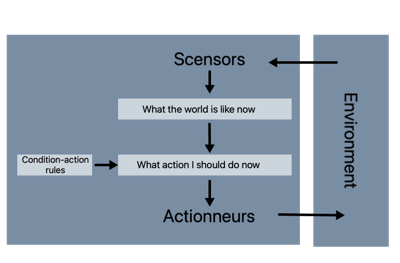Figure 2

The most simplistic agents are simply "reflex" agents, i.e. agents that have a set of internally enacted rules that they follow in an absolute way, as depicted above.

In chess, this would be like recording all possible moves for all board configurations and choosing the best move.

The reflex agents are limited to simpler cases like that of an automatic vacuum cleaner: if there is dust under the vacuum cleaner, do the action of vacuuming, if there is dust elsewhere, do the action of moving, otherwise return the vacuum cleaner to the charging station.

Two natural evolutions (which can be combined together) of these agents have emerged.

The first, which we will not dwell on here, is the notion of logical agents. Like reflex agents, logical agents also have a set of encoded rules. However, logical agents do not carry rules on a case-by-case basis. The agent's rules are reasoning rules, it also carries an "internal representation of the world". By combining information about the world, information about the current state of its immediate environment, the logical agent will automatically design the solution.

Referring back to the analogy of the chess game, rather than stipulating "when the Queen is on g1, the enemy King on g8 and the Bishop on h6, play the Queen on g7" and all other cases, we simply integrate into the agent's process "if victory is possible at the next turn, proceed with the movement to checkmate", and let the agent calculate if a checkmate is indeed likely in the possible environment (the state of the board) knowing the information of the world (the rules of the game of the game, especially the movement of the pieces).

The second is a learning agent. Let us consider figure 3. Although the "performance" part remains more or less the same (a set of rules to follow), these rules are modified by the examples that the system encounters.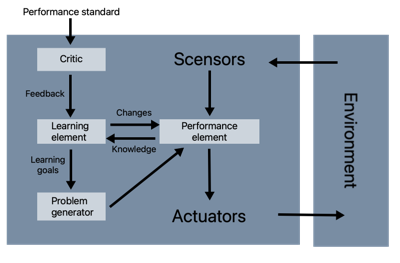Figure 3

We therefore encounter three new blocks here:

• the learning block, responsible for improving the rules,
• a criticism block that allows the agent to get feedback on the rules implemented and
• a problem generation block that allows the objectives to be modified to find a balance between (1) meeting the agent's initial need and (2) discovering relevant information.

Let's illustrate with the image of a robot equipped with a compass and provided arrival coordinates. Initially the robot will move straight towards the goal, but if it encounters an obstacle, the problem generator will define additional goals, such as turning 90 degrees, to obtain information and possibly find a new path.

## Machine learning and data mining

As we have just seen, artificial intelligences can be built in the form of learning agents. And who says learning agent says machine learning (ML).

Machine learning is a discipline that, despite the mysteries that surround it, simply extends probabilistic and statistical approaches to geometric problems.

The two largest classes of ML are supervised and unsupervised learning. To better understand, let's use sets of points in space.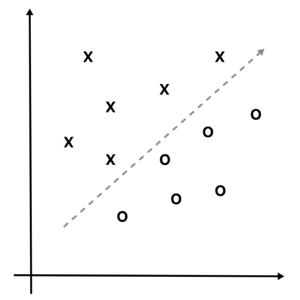Figure 4

The supervised context corresponds to the graph on the left, where the points have distinct labels "x" and "o" (for example, the observations related to the language Customers are speaking). In such a case, the goal of an ML system is to create a function (draw a line for example) separating the two categories. This function, which is called a model, allows to make posteriori predictions for new incoming data: Essentially by comparing its position in the model to determine the most probable category of its labeling ("o" or "x").Figure 5

In an unsupervised application, no feature is considered as prevailing over the others, meaning that there is no learning label, all points are in the same category, as depicted in the graph on the right. In such a case, one of the possible approaches is that the machine will create groups using the proximity of the points. We can imagine, on this second graph, three groups, the first one taking the 4 points at the bottom right, the second one the 4 points at the bottom left and the last one the 4 points at the top.

Whether the learning is supervised or not, it relies first and foremost on the discovery of regularities in the data (for example, a position in space). Data mining is the domain that encompasses algorithms that allow the discovery of regularities.

To summarize, we can say that: data mining is the domain that aims at extracting regularities from a data set, machine learning is the field that, from regularities discovered in the data, aims at editing action rules for a system and artificial intelligence is the field that allows the creation of rational agents that can use ML.

The three disciplines are independent although strongly related.

## Neural networks and deep learning

At the moment, there is often confusion between "artificial intelligence" and "deep learning" (DL). To explain to the uninitiated to the notion of DL we have to start by presenting what is a neural network and a neuron. Of course, we make here an introductory presentation without exposing the mathematics behind it, so for more advanced readers, the book1 is the reference to get.

#### Artificial neural network

The artificial neuron, also called formal neuron, can be seen as a regression. Let’s illustrate the diagram of a neuron in figure 5 to explain the principle. A neuron, like a regression, can be considered as a supervised learning system.

For example, in the case of the graph in Figure 4, the neuron will learn the characteristics (angle and position) of the line separating the "x" from the "o", and then use this line to predict the class of any new input like any ML system.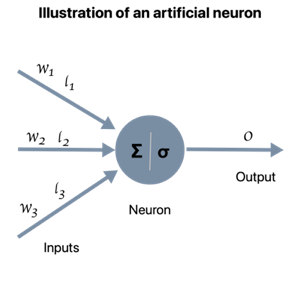Figure 6

The neuron is composed of several parts:

• a set of entries, which correspond to the coordinates of the points to consider. For example, one input corresponds to the age and the other input to the capital of a client
• these inputs are connected by the arrows on the left to the neuron. By passing through the arrows, the inputs are multiplied by a coefficient specific to each arrow. The coefficients play the role of marker of the importance of the input, for example if the capital of a customer is 9 times more important than his age to decide if he is English or French speaking, the coefficient of the capital would be 0.9 while that of the age 0.1. Finally, the set of coefficients allows us to define the equation of a line that serves as a model.
• The left part of the neuron, the summation, sums up the inputs (after multiplication by the importance). This allows to obtain a number reflecting the relative position of the input point (the position of the client's point) with respect to the line internalized by the neuron (below or above)
• the right part of the neuron is what we call the activation function. This is the function that, taking into account the relative position indicated, will assign the final class ("x" or "o") to the input provided. The activation functions can be simple (for example, if the point is above the right put an "x" otherwise an "o") or probabilistic (for example, the closer the point is to the right the closer the score provided is to 50-50 and the further up it is the closer it is to 100-0).

During the learning phase, the neuron tests its model (the choice of coefficients defining the line separating "x" and "o") for all known points and compares the expected result with its prediction. At each error, the coefficients are adjusted until the perfect line is found (or, failing that, the one that allows the least errors). The advantage of not visualizing the neurons mathematically as regressions but as in figure 5 is that it becomes easy to connect neurons to the chain: the output of one neuron is sent to the input of another. Such a construction is called a neural network as shown in figure 6.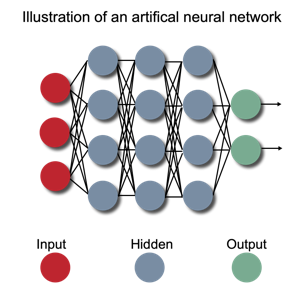Figure 7

#### Deep learning

Deep learning (DL) is simply the application domain of so-called deep neural networks. In figure 6, we can see that some neurons are directly connected to the real inputs, some are directly connected to the outputs and others are between the inputs and outputs, only connected to other neurons, on one side or the other. These neurons are called "hidden". What characterizes the DL is the depth of the network, in other words the number of steps (of transformations) in the hidden layers that an input signal must make to reach the output (here three). We consider a network as deep as soon as there is at least one hidden layer (some will argue for two or three layers, but the idea remains the same). The interest of this depth is the possibility to combine the characteristics of the inputs in a hierarchical way. Let's imagine an application case of a network detecting on 1080 pixels images if it is a fork or a human being that is represented. The input layer will integrate the 1080 pixels, the first layer will detect straight line segments (each neuron will be responsible for testing its own segment), the second layer will detect angles and curves (each neuron will have a different angle or curve), the next layer will have more recognizable features (nose, sleeve, mouth, teeth, ...) and finally the output layer instead of receiving the image will receive a set of features (as the image has a nose and a mouth) and then will be able to take the decision.

## Conclusion

As we have seen, AI is a vast field that can rely on machine learning, which itself relies on the use of regularities discovered by data mining algorithms. Machine learning is a vast field containing many techniques including deep learning, a field built on neural networks with many hidden layers. Hoping to have enlightened you!

Références:

1 Yoshua Bengio, Ian Goodfellow, and Aaron Courville. Deep learning, volume 1. MIT press Massachusetts, USA:, 2017.
2 Nils J Nilsson. The quest for artificial intelligence. Cambridge University Press, 2009.
3 Stuart Russell and Peter Norvig. Artificial intelligence: a modern approach. 2002.